Next: The model Up: Isospin-breaking corrections to superallowed Previous: Isospin-breaking corrections to superallowed

# Introduction

By studying isotopes with enhanced sensitivity to fundamental symmetries, nuclear physicists can test various aspects of the Standard Model in ways that are complementary to other sciences. For example, a possible explanation for the observed asymmetry between matter and anti-matter in the universe could be studied by searching for a permanent electric dipole moment larger than Standard Model predictions in heavy radioactive nuclei that have permanent octupole shapes. Likewise, the superallowed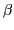-decays of a handful of rare isotopes with similar numbers of protons and neutrons, in which both the parent and daughter nuclear states have zero angular momentum and positive parity, are the unique laboratory to study the strength of the weak force.

What makes these pure vector-current-mediated (Fermi) decays so useful for testing the Standard Model is the hypothesis of the conserved vector current (CVC), that is, independence of the vector current on the nuclear medium. The consequence of the CVC hypothesis is that the product of the statistical rate function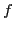and partial half-life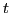for the superallowed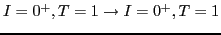Fermi-decay should be nucleus independent and equal to: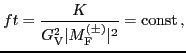(1)

where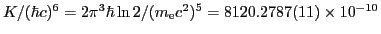GeV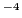s is a universal constant;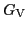stands for the vector coupling constant for semi-leptonic weak interaction, and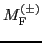is the nuclear matrix element of the isospin rising or lowering operator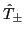.

The relation (1) does not hold exactly and must be slightly amended by introducing a set of radiative corrections to the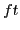-values, and a correction to the nuclear matrix element due to isospin-symmetry breaking: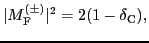(2)

see Refs. [1,2,3,4,5] and references cited therein. Since these corrections are small, of the order of a percent, they can be approximately factorized and arranged in the following way: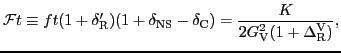(3)

with the left-hand side being nucleus independent. In Eq. (3),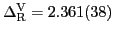% stands for the nucleus-independent part of the radiative correction ,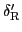is a transition-dependent (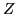-dependent) but nuclear-structure-independent part of the radiative correction [6,3], and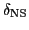denotes the nuclear-structure-dependent part of the radiative correction [7,3].

In spite of theoretical uncertainties in the evaluation of the radiative and isospin-symmetry-breaking corrections, the superallowed-decay is the most precise source of experimental information for determining the vector coupling constant, and provides us with a stringent test of the CVC hypothesis. In turn, it is also the most precise source of the matrix element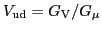of the Cabibbo-Kobayashi-Maskawa (CKM) three-generation quark mixing matrix [8,9,3,10]. This is so because the leptonic coupling constant,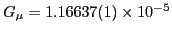GeV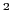, is well known from the muon decay .

The advantage of the superallowed-decay strategy results from the fact that, within the CVC hypothesis,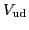can be extracted by averaging over several transitions in different nuclei. For precise tests of the Standard Model, only these transitions that have-values known with a relative precision better than a fraction of a percent are acceptable. Currently, 13 canonical" transitions spreading over a wide range of nuclei from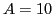to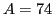meet this criterion (have-values measured with accuracy of order of 0.3% or better) and are used to evaluate the values ofand.

In this work we concentrate on the isospin-breaking (ISB) corrections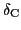, which were already computed by various authors, using a diverse set of nuclear models [11,3,12,13,14,15,16,17,]. The standard in this field has been set by Towner and Hardy (HT)  who used the nuclear shell-model to account for the configuration mixing effect, and the mean-field (MF) approach to account for a radial mismatch of proton and neutron single-particle (s.p.) wave functions caused by the Coulomb polarization. In this study, which constitutes an extension of our earlier work , we use the isospin- and angular-momentum-projected density functional theory (DFT). This method can account, in a rigorous quantum-mechanical way, for spontaneous symmetry-breaking (SSB) effects, configuration mixing, and long-range Coulomb polarization effects.

Our paper is organized as follows. The model is described in Sec. 2. The results of calculations for ISB corrections to the superallowed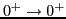Fermi transitions are summarized in Sec. 3. The ISB corrections to the Fermi matrix elements in mirror-symmetric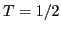nuclei are discussed in Sec. 4. Section 5 studies a particular case of the Fermi decay ofCl. Finally, the summary and perspectives are given in Sec. 6.Next: The model Up: Isospin-breaking corrections to superallowed Previous: Isospin-breaking corrections to superallowed
Jacek Dobaczewski 2012-10-19